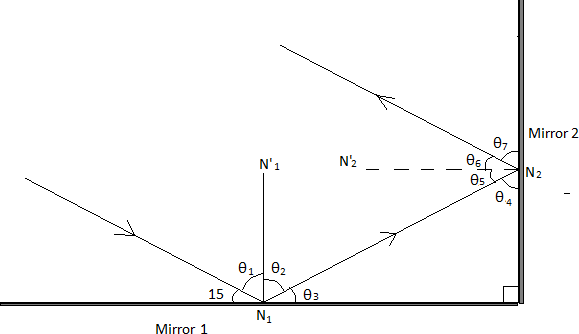# LAW OF REFLECTION(PHYSICS)

Introduction

Physical sciences

Topic: Optics(law of reflection)

Here is a quick rundown of what we will be working on:

Reflection is when light changes direction by bouncing off a surface.

When light is reflected off a mirror, it hits the mirror at the same angle (θi, the incidence angle) as it reflects off the mirror (θr, the reflection angle).

The normal is an imaginary line which lies at right angles to the mirror where the ray hits it.                                                                              .1. State the law of reflection.
2. What happens to a ray of light when it reaches:                                                                                                                                    (a) a mirror?                                                                                                                                                                                           (b) a lens?
3. Two flat mirrors are perpendicular to each other. An incoming beam of light makes an angle of 15° with the mirror 1, continues to mirror 2 and is reflected as shown in Figure 1. N1’N1 and N2’N2 are the normal to mirror 1 and mirror 2 respectively.Figure. 1

a). Calculate angles θ1 to θ3

b). Calculate angles θ4 to θ7

4. Explain whether or not the path of the ray incident to mirror 1 is parallel to the path of the reflected ray from mirror 2.

Process

Step 1:

Learn about the Law of reflection

https://phys.libretexts.org/Courses/University_of_California_Davis/UCD%3A_Physics_7C_-_General_Physics/09%3A_Optics/9.2%3A_Optics_and_Images/9.2.2%3A_Reflection

Step 2:

Watch this video for assistance on how to do the calculations

Step 3:

Evaluation

When doing your work make use of this mark allocation as your guide of how many marks are on each question.

Questions Marks
1 3
2(a) 2
2(b) 2
3(a) 6
3(b) 8
4 4

Total marks: 
The memorandum will be made available after submission.

Conclusion